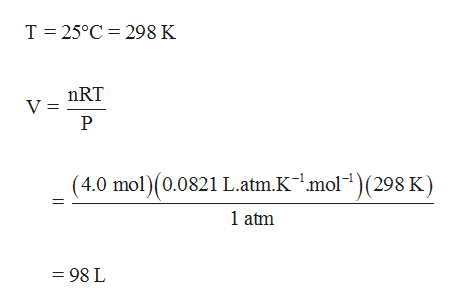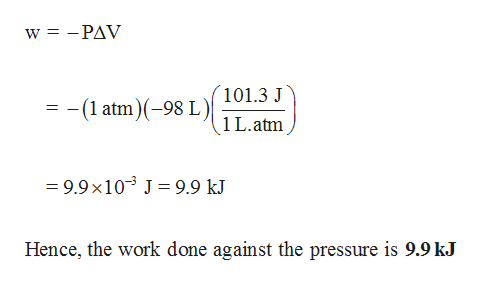# Be sure to answer all parts.Consider the reactionДН-92.6 kJ/molN2(g)+3H2(g) 2NH3(g)rxnIf 2.0 moles of N2 react with 6.0 moles of H2 to form NH3, calculate the work done (in joules) against apressure of 1.0 atm at 25°C.JWhat is AU for this reaction? Assume the reaction goes to completion.AU=kJ

Question
297 viewshelp_outlineImage TranscriptioncloseBe sure to answer all parts. Consider the reaction ДН -92.6 kJ/mol N2(g)+3H2(g) 2NH3(g) rxn If 2.0 moles of N2 react with 6.0 moles of H2 to form NH3, calculate the work done (in joules) against a pressure of 1.0 atm at 25°C. J What is AU for this reaction? Assume the reaction goes to completion. AU= kJ fullscreen
check_circle

Step 1

Two moles of N2 reacts with six moles of hydrogen giving six moles of ammonia. Initial number of moles present is eight (two moles of nitrogen and six moles of hydrogen). Product obtained is four moles of ammonia; hence there is a net loss of four moles of gas.

Change in volume can be calculated from change in moles using Ideal gas equation as follows,help_outlineImage TranscriptioncloseT 25°C 298 K nRT V = (4.0 mol)(0.0821 L.atm.Kmol)(298 K) 1 atm =98 L fullscreen
Step 2

The number of moles gets decreased during the reaction (compression), the value of ΔV ...help_outlineImage Transcriptionclosew =-PAV 101.3 J (1 atm)(-98 L) 1 L.atm =9.9 x103 J9.9 kJ Hence, the work done against the pressure is 9.9 kJ fullscreen

### Want to see the full answer?

See Solution

#### Want to see this answer and more?

Solutions are written by subject experts who are available 24/7. Questions are typically answered within 1 hour.*

See Solution
*Response times may vary by subject and question.
Tagged in

### Chemical Thermodynamics